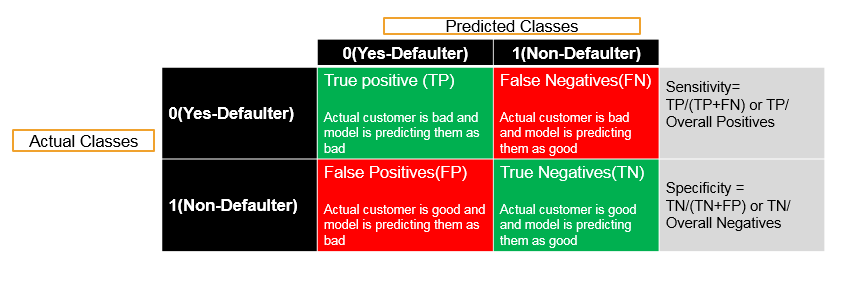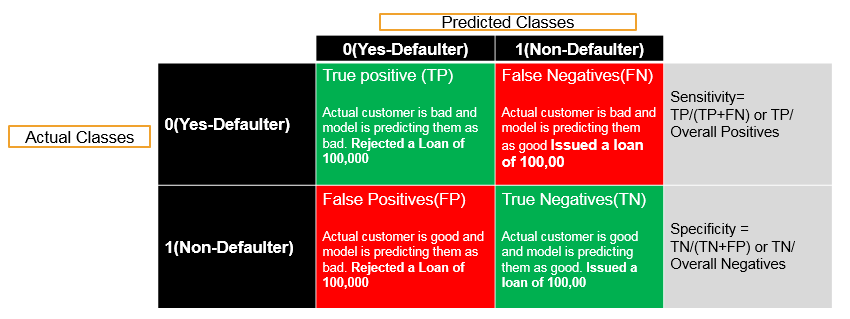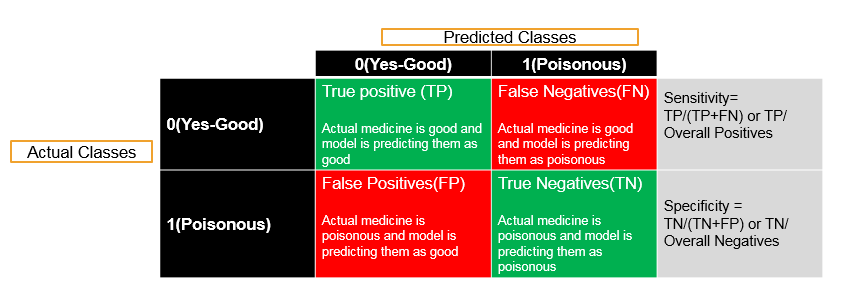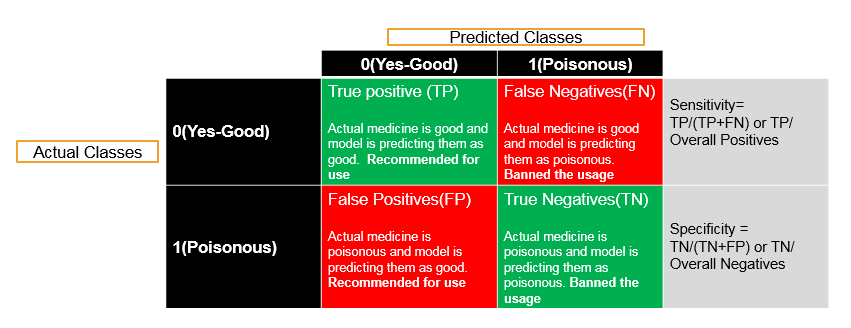• No products in the cart.

# 204.4.2 Calculating Sensitivity and Specificity in Python

##### Building a model, creating Confusion Matrix and finding Specificity and Sensitivity.

Link to the previous post: https://statinfer.com/204-4-1-model-section-and-cross-validation/

This post is an extension of the previous post. Here, we will look at a way to calculate Sensitivity and Specificity of the model in python.

## Calculating Sensitivity and Specificity

### Building Logistic Regression Model

In :
#Importing necessary libraries
import sklearn as sk
import pandas as pd
import numpy as np
import scipy as sp

In :
#Importing the dataset
###to see head and tail of the Fiber dataset

Out:
active_cust income months_on_network Num_complaints number_plan_changes relocated monthly_bill technical_issues_per_month Speed_test_result
0 0 1586 85 4 1 0 121 4 85
1 0 1581 85 4 1 0 133 4 85
2 0 1594 82 4 1 0 118 4 85
3 0 1594 82 4 1 0 123 4 85
4 1 1609 80 4 1 0 177 4 85
In :
#Name of the columns/Variables
Fiber_df.columns

Out:
Index(['active_cust', 'income', 'months_on_network', 'Num_complaints',
'number_plan_changes', 'relocated', 'monthly_bill',
'technical_issues_per_month', 'Speed_test_result'],
dtype='object')
In :
#Building and training a Logistic Regression model
import statsmodels.formula.api as sm
logistic1 = sm.logit(formula='active_cust~income+months_on_network+Num_complaints+number_plan_changes+relocated+monthly_bill+technical_issues_per_month+Speed_test_result', data=Fiber_df)
fitted1 = logistic1.fit()
fitted1.summary()

Optimization terminated successfully.
Current function value: 0.493647
Iterations 9

Out:
Dep. Variable: No. Observations: active_cust 100000 Logit 99991 MLE 8 Fri, 18 Nov 2016 0.2748 19:16:40 -49365 True -68074 0
coef std err z P>|z| [95.0% Conf. Int.] -17.6101 0.301 -58.538 0.000 -18.200 -17.020 0.0017 8.21e-05 20.820 0.000 0.002 0.002 0.0288 0.001 28.654 0.000 0.027 0.031 -0.6865 0.030 -22.811 0.000 -0.746 -0.628 -0.1896 0.008 -24.940 0.000 -0.205 -0.175 -3.1626 0.040 -79.927 0.000 -3.240 -3.085 -0.0022 0.000 -13.995 0.000 -0.003 -0.002 -0.3904 0.007 -54.581 0.000 -0.404 -0.376 0.2222 0.002 93.435 0.000 0.218 0.227
In :
###predicting values
predicted_values1=fitted1.predict(Fiber_df[["income"]+['months_on_network']+['Num_complaints']+['number_plan_changes']+['relocated']+['monthly_bill']+['technical_issues_per_month']+['Speed_test_result']])
predicted_values1[1:10]

Out:
array([ 0.83701059,  0.83271114,  0.83117449,  0.80896979,  0.8520262 ,
0.82713018,  0.85504571,  0.85131352,  0.85537857])
In :
### Converting predicted values into classes using threshold
threshold=0.5

predicted_class1=np.zeros(predicted_values1.shape)
predicted_class1[predicted_values1>threshold]=1
predicted_class1

Out:
array([ 1.,  1.,  1., ...,  1.,  1.,  1.])
In :
#Confusion matrix, Accuracy, sensitivity and specificity
from sklearn.metrics import confusion_matrix

cm1 = confusion_matrix(Fiber_df[['active_cust']],predicted_class1)
print('Confusion Matrix : \n', cm1)

total1=sum(sum(cm1))
#####from confusion matrix calculate accuracy
accuracy1=(cm1[0,0]+cm1[1,1])/total1
print ('Accuracy : ', accuracy1)

sensitivity1 = cm1[0,0]/(cm1[0,0]+cm1[0,1])
print('Sensitivity : ', sensitivity1 )

specificity1 = cm1[1,1]/(cm1[1,0]+cm1[1,1])
print('Specificity : ', specificity1)

Confusion Matrix :
[[29492 12649]
[10847 47012]]
Accuracy :  0.76504
Sensitivity :  0.699841009943
Specificity :  0.812527005306


#### Changing Threshold to 0.8

In :
### Converting predicted values into classes using new threshold
threshold=0.8

predicted_class1=np.zeros(predicted_values1.shape)
predicted_class1[predicted_values1>threshold]=1
predicted_class1

Out:
array([ 1.,  1.,  1., ...,  1.,  1.,  1.])

#### Change in Confusion Matrix, Accuracy and Sensitivity-Specificity

In :
#Confusion matrix, Accuracy, sensitivity and specificity
from sklearn.metrics import confusion_matrix

cm1 = confusion_matrix(Fiber_df[['active_cust']],predicted_class1)
print('Confusion Matrix : \n', cm1)

total1=sum(sum(cm1))
#####from confusion matrix calculate accuracy
accuracy1=(cm1[0,0]+cm1[1,1])/total1
print ('Accuracy : ', accuracy1)

sensitivity1 = cm1[0,0]/(cm1[0,0]+cm1[0,1])
print('Sensitivity : ', sensitivity1 )

specificity1 = cm1[1,1]/(cm1[1,0]+cm1[1,1])
print('Specificity : ', specificity1)

Confusion Matrix :
[[37767  4374]
[30521 27338]]
Accuracy :  0.65105
Sensitivity :  0.896205595501
Specificity :  0.472493475518

### Sensitivity and Specificity

• By changing the threshold, the good and bad customers classification will be changed hence the sensitivity and specificity will be changed.
• Which one of these two we should maximize? What should be ideal threshold?
• Ideally we want to maximize both Sensitivity & Specificity. But this is not possible always. There is always a trade-off.
• Sometimes we want to be 100% sure on Predicted negatives, sometimes we want to be 100% sure on Predicted positives.
• Sometimes we simply don’t want to compromise on sensitivity sometimes we don’t want to compromise on specificityThe threshold is set based on business problem

### When Sensitivity is a High Priority

• Predicting a bad customers or defaulters before issuing the loan• Predicting a bad defaulters before issuing the loan• The profit on good customer loan is not equal to the loss on one bad customer loan.
• The loss on one bad loan might eat up the profit on 100 good customers.
• In this case one bad customer is not equal to one good customer.
• If p is probability of default then we would like to set our threshold in such a way that we don’t miss any of the bad customers.
• We set the threshold in such a way that Sensitivity is high.
• We can compromise on specificity here. If we wrongly reject a good customer, our loss is very less compared to giving a loan to a bad customer.
• We don’t really worry about the good customers here, they are not harmful hence we can have less Specificity.

### When Specificity is a High Priority

• Testing a medicine is good or poisonous• Testing a medicine is good or poisonous• In this case, we have to really avoid cases like , Actual medicine is poisonous and model is predicting them as good.
• We can’t take any chance here.
• The specificity need to be near 100.
• The sensitivity can be compromised here. It is not very harmful not to use a good medicine when compared with vice versa case.

### Sensitivity vs Specificity – Importance

• There are some cases where Sensitivity is important and need to be near to 1.
• There are business cases where Specificity is important and need to be near to 1.
• We need to understand the business problem and decide the importance of Sensitivity and Specificity.

The next post is about roc and auc.

Link to the next post : https://statinfer.com/204-4-4-roc-and-auc/

24th January 2018

### 2 responses on "204.4.2 Calculating Sensitivity and Specificity in Python"

1.Thanks very informative blog, well done! I believe there is a smallish typo within the calculations for the metrics though. Note that the confusion matrix evaluates to:
[TN FP]
[FN TP]

Hence, for example, metrics for specificity should be cm1[0,0]/(cm1[0,0]+cm1[1,0]). Similarly for the other metrics on here.

2.— sorry, meant to write cm1[0,0]/(cm1[0,0]+cm1[0,1]) for specificity 😉

Statinfer Software Solutions LLP

Software Technology Parks of India,
NH16, Krishna Nagar, Benz Circle,